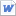검색어 입력폼

Biosystems control and lab 3

저작시기 2014.02 |등록일 2017.03.25MS 워드 (docx) | 7페이지 | 가격 2,000원

목차

Ⅰ. Introduction
Ⅱ. Procedures and results
Ⅲ. Conclusion

본문내용

In this lab, we used the servomechanism in this lab. The servomechanism is the branch of control that regulates input value by using position control. That is, the higher the position value, the bigger the input value. And we used velocity feedback to control the response of the system. Increasing velocity feedback increases the stability of the system.

<중 략>

In this experiment, First, setting controller Servo, signal step, level 20%, offset 0%, rate 10msec, Reference External, controller gain Kc(Proportional controller gain) 0.8 and VFB(velocity feeback) Off. After that, we rotated the command potentiometer between 30 and 180 degrees and watched the output dial follow. We regulated input voltage by using the Command Potentiometer. The result is Fig.2. And we changed the Kc to 2.5 and 5. The results are in Fig.3 (Kc=2.5) and Fig.4 (Kc=5.0) In these results, we can see that when the Kc is increased, the response of the system is improved, but stability is decreased.

없음